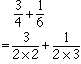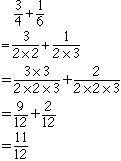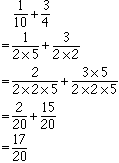Subject: ADDING FRACTIONS In adding fractions, how do I rewrite the fractions so that the denominators are equal? The problem is 3/4 + 1/6 = The other problem is 11/18 + 4/9 = Thanks for your help. I am a Grade 6 student. Hi, First you should find the prime factors of each of the denominators. 4 = 2 x 2 6 = 2 x 3 Now construct the common denominator. Begin by writing the denominator of the first fraction. 2 x 2 Next look at the second denominator and see if there is anything you haven't included. In your problem the next denominator is 2 x 3. You already have a 2 (in fact you have two of them) but you don't have a 3 so update your common denominator construction by multiplying by 3. 2 x 2 x 3 This is the common denominator, 2 x 2 x 3 = 12. Finally you need to add the fractions.To change the denominator of the first fraction to the common denominator, 12, you need to multiply it by 3. Thus multiply both the numerator and denominator of the first fraction by 3. To change the denominator of the second fraction to 12 you need to multiply by 2. Thus multiply the numerator and denominator of the second fraction by 2.Let try another, 1/10 + 3/4. Write each denominator in terms of its prime factors. 10 = 2 x 5 and 4 = 2 x 2 Now construct the common denominator. Start with the denominator of the first fraction. 2 x 5 From the second denominator you need another 2, thus the common denominator is 2 x 2 x 5 = 20 Finally add the fractions.I hope this helps, Penny Go to Math Central